# Welcome! Have a look at my research!

## EEG/ERP rounding event latencies

22.10.2019 Edit: Thanks Matt Craddock, I understand the source of the problem better. He mentioned that this should not occur if the amplifiers record the triggers as trigger channels (before converting it to events). And mentions that this could happen through downsampling. Indeed after checking in the dataset I used it was downsampled from 1024 to 512Hz. This made many eventlatencies ~ X.50001, which will be uprounded with round and floored with floor. This gives some context to the problem.

TLDR; EEGlab allows for non-integer event latencies (in units of samples). Eeglab chose floor(latency), while others e.g. unfold & fieldtrip choose round(latency) to round the latency to samples. This leads to differences between toolboxes, in my example of up to 1.5µV (or ~25% ERP magnitude). Importantly, this probably does not introduce bias between conditions

This is an ERP, actually its two ERPs. One is calculated using the “unfold” toolbox and one using eeglab’s pop_epoch function

If you look very closely, you can see that they are not identical, even though they should be. So – whats the difference?

It turns out that EEGlab saves event latencies in samples (e.g. stimulus is starting at sample 213), but also allows non-integer latencies (e.g. stimulus is starting between 212 and 213, to be exact: at sample 212.7). This makes sense, i.e. if your EEG sampling resolution is 100Hz you might know your stimulus onset with higher precision and not in 10ms bins. But in order to get ERPs we have to “cut” the signal at the event onset. EEGlab uses “floor” for this and rounds the stimulus onset from 212.7 to sample 212. Other toolboxes (unfold / fieldtrip) use “round”, thus the event would start at sample 213.

It turns out that in the example you see above, this introduces a difference between the two ERPs of 0.5µV (!) thats around 8% of the magnitude.

This is just a random example I stumbled upon. With lower sampling rates, this effect should increase. Indeed, downsampling to 128 Hz gives us a whopping difference of 1.5µV.

#### Floor vs. round (vs. others?)

The benefit of floor, at least the one I can think of, is that it would never shift the onset of a stimulus in the future. That is, it is causal. Possibly there are other benefits I am not aware of.

The benefit of round is that it more accurately reflects the actual stimulus onset. Possibly there are other benefits I am not aware of

Given that we mostly use acausal filtering anyway, I think the causal benefit is not very strong.

There is yet another alternative: a weighted average between samples. We could “split” the event onset to two samples, i.e. if we want the instantaneous stim-onset response, and stim onset is at sample 12.3, then sample 12 should be weighted 30% and sample 13 by 70%. I have to explore this idea a bit more, but I think its very easy to implement in unfold and test. But this for a new blogpost.

## The big picture

In the fMRI community there are papers from time to time reporting that different analysis tools (or versions) lead to different results. I am not aware of any such paper in the EEG community (if you know one, let me know please!) but I think it would be nice if somebody would do such comparisons.

I currently do not forsee if such an event-latency-rounding difference could possibly introduce bias in condition differences. But I forsee that changing it will be difficult for the EEGlab developers, as “floor” has been around for a very long time in eeglab.

## Code

Note that I did not use simulation here (but could have, it should be straight) but I cannot publicly share the data at this point in time.

load('EEG_subj8_inkl_ufresult.mat')
%%
%EEG = pop_resample(EEG,128);
timelimits = [-.3 .5];
EEG = uf_designmat(EEG,'eventtypes','stimulus','formula','y~1');
%deconv based "round" analysis
EEG = uf_timeexpandDesignmat(EEG,'timelimits',timelimits);
EEG = uf_glmfit(EEG,'channel',63);

%eeglab based "floor" analysis
EEGe = pop_epoch(EEG,{'stimulus'},timelimits);

ufresult = uf_condense(EEGe);
%% ERP plot
figure
plot(ufresult.times,mean(EEGe.data(63,:,:),3)) %floor
hold on
plot(ufresult.times,ufresult.beta(63,:,1)) %round
xlim(timelimits)
xlabel('time [s]')
ylabel('ERP [µV]')
box off
export_fig erp.png -m3 -transparent
%% difference plot
figure
title('Diff "floor"-"round"')
plot(ufresult.times,mean(EEGe.data(63,:,:),3)-ufresult.beta(63,:,1)) %floor-round
xlim(timelimits)
xlabel('time [s]')
ylabel('ERP [µV]')
box off
export_fig diff.png -m3 -transparent


## EEG / ERP Lecture

I just gave my lecture at the Donders Toolkit. Many cool and interesting questions! I wish I had two hours, I had to skip some things I was excited about in favour of solid basics.

Find the slides here as pdf (5 mb) or pptx (23 mb).

In case you are interested in other EEG slides, here are slides on overlap correction (deconvolution) and non-linear modeling (pptx, 8mb), an introduction to linear models (pptx, 50mb), and slides on multiple comparison corrections (pptx, 5mb)

Can’t give a proper license unfortunately, as some slides are based on old Donder Toolkit Slides, handed down from the years. All other authors I stole slides should be acknowledge, hope I did not forget something. Do as you wish with the slides. May the copywrite gods see favourable on our educator souls

## Electrode drift in EEG

I coudn’t find an image of electrode drift for my slides, so here I quickly generated one. The only fancy thing is the usage of datetime to have minutes on the x-axis (I also made this post so I don’t forget this trick ;))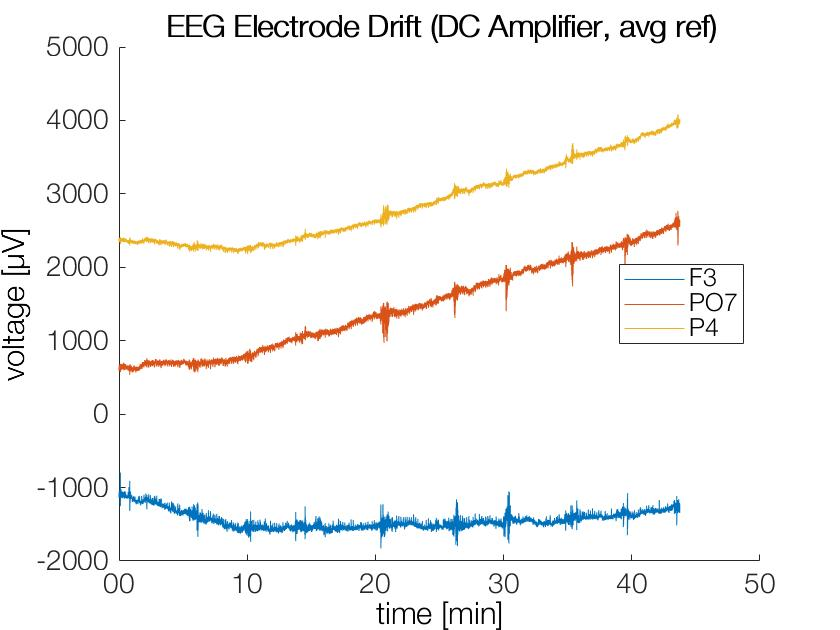DC-Amplifier (REFA-2, tmsi) plot of three electrodes over ~45min recording data. Strong DC-Offsets and drifts are observed (this is typical & normal)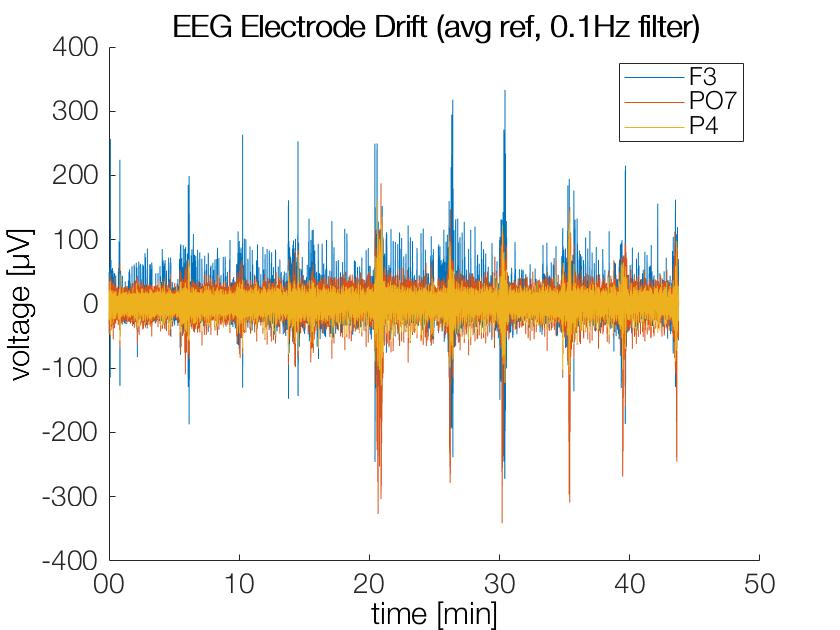After filtering at 0.1Hz the strong drifts and offsets are gone (as intended), the y-axis has a very different axis now!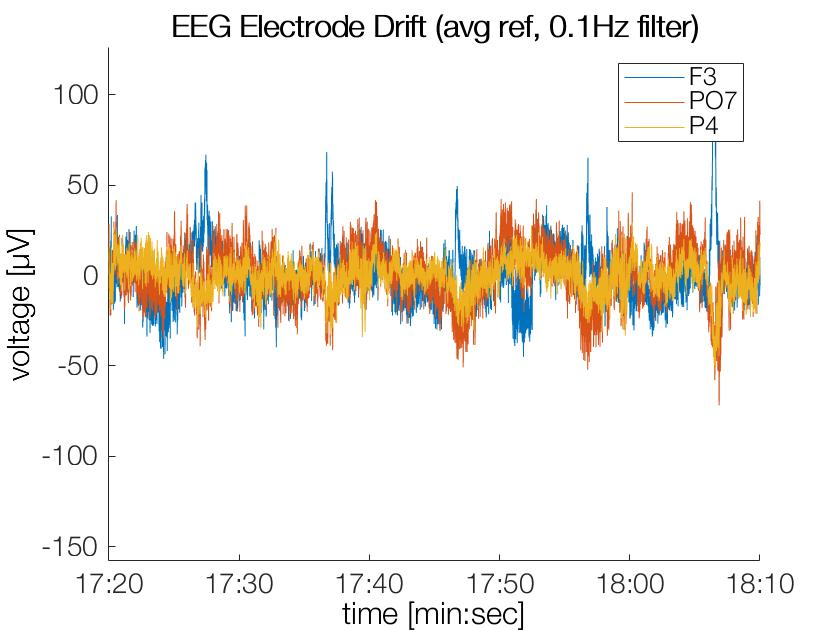Obviously a cutoff-freq of 0.05Hz in the filter will still allow for some slow moving drifts. These offsets are usually accomodated for by baseline corrections

Thanks to Anna Lisa Gert for this dataset

% Load Data
% Load filtered data (takes 35min to filter...)
%%
% Convert time to actual time
timesnew = datetime(EEG.times/1000,'ConvertFrom','epochtime','Epoch','2000-01-01');
% select random channels
chix = [5,63,27];

% Plot the unfiltered data
plot(timesnew,EEG.data(chix,:)')
% Make the plot beautiful
datetick('x','MM','keeplimits','keepticks') % only show minutes
xlabel('time [min]')
ylabel('voltage [µV]')
legend({EEG.chanlocs(chix).labels},'Location','East')
box off
title('EEG Electrode Drift (DC Amplifier, avg ref)')
set(gca,'fontsize', 14) % for a presentation
set(gca, 'FontName', 'HelveticaNeueLT Pro 45 Lt')
%%
% Convert again because data have been resampled
timesnew = datetime(EEG_filt.times/1000,'ConvertFrom','epochtime','Epoch','2000-01-01');

plot(timesnew,EEG_filt.data(chix,:)')

datetick('x','MM','keeplimits','keepticks')
xlabel('time [min]')
ylabel('voltage [µV]')
legend({EEG.chanlocs(chix).labels})
box off
title('EEG Electrode Drift (avg ref, 0.1Hz filter)')
set(gca,'fontsize', 14) % for a presentation
set(gca, 'FontName', 'HelveticaNeueLT Pro 45 Lt')


## A (short) laypersons summary

TLDR; Using our data/paper you can find out how well your eye tracker is doing in measuring your favourite eye movement

This newly published paper is open access and I share first co-author ship with the amazing Katharina Groß! In addition this project was done together with Inga Ibs and Peter König: A new comprehensive eye-tracking test battery concurrently evaluating the Pupil Labs glasses and the EyeLink 1000

We move our eyes about four times per second, which is more often than our heart beats! Many studie show that these eye movements are a window into our minds (e.g. König et al. 2017) and are commonly used for basic research, marketing research and clinical assesments.

Even though eyetrackers are so commonly used and so powerful, they are rarely tested for how well they perform besides the manufacturers. Even more critical, no test battery existed before we set out on this project. Together with Katharina Groß (and Inga Ibs and Peter König) we developed a new eye tracker test battery which included most of the typically studied eye movements.

We chose to test two popular eye trackers concurrently: The Eyelink 1000 and the Pupil Labs glasses. The former is the working horse of eye movement research (release 2005), the latter the open hardware/source innovator (release 2014).

Our study reveals some strengths and some weakenesses for both eye trackers, but also of our newly proposed test battery. The results are numerours, so I will only depict two tasks:

### Free Viewing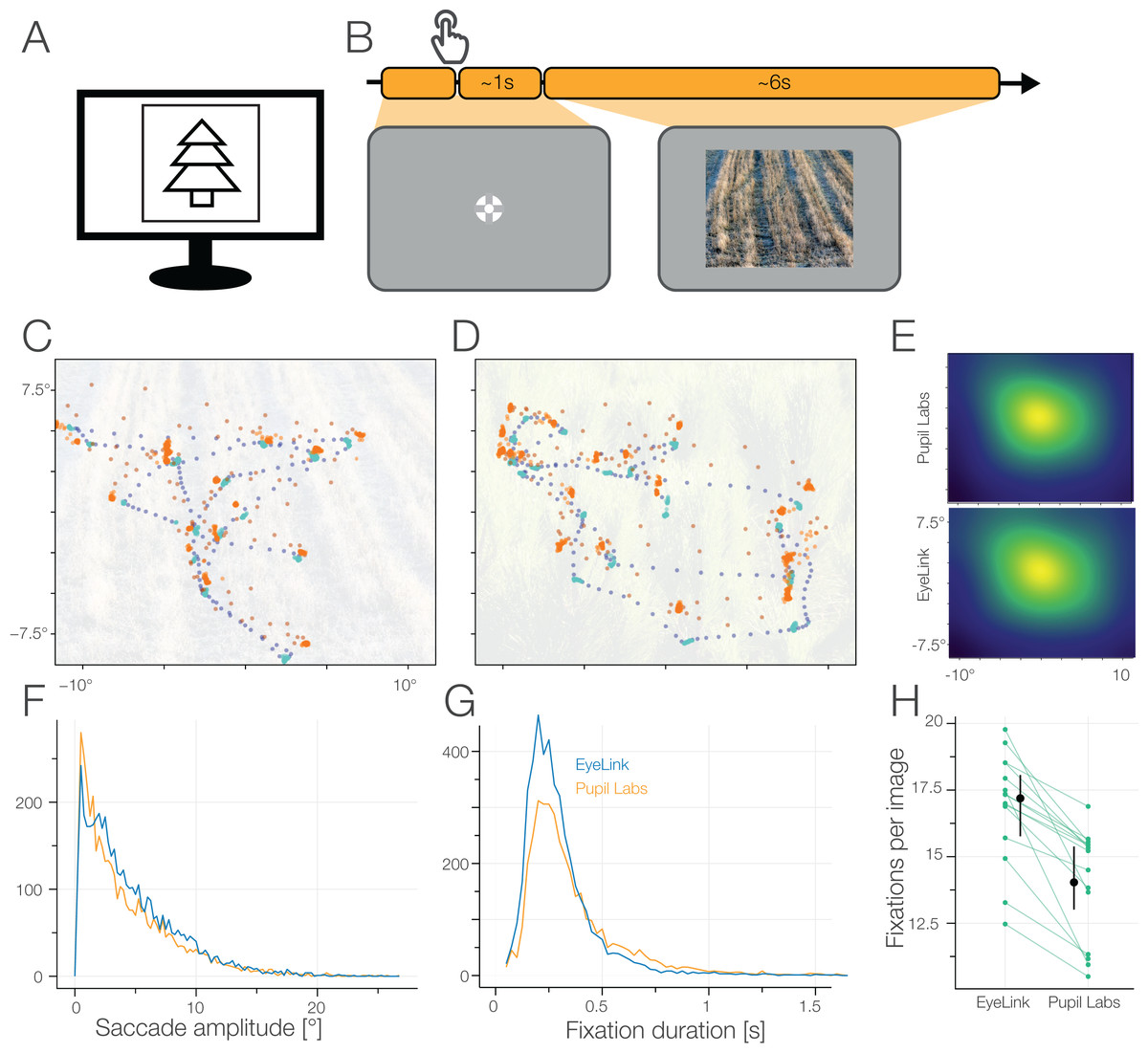Here you can see where a participant is looking on an image (C & D) with the task to simply explore the image. You can clearly see that the eye trackers agree on the coarse scale (blue & red dots kind of overlay), but not in the specific details (e.g. disagreements that worse/better at some locations. Also the red (pupil labs) eye-tracker has much higher variability during eye movements .

### Pupil Dilation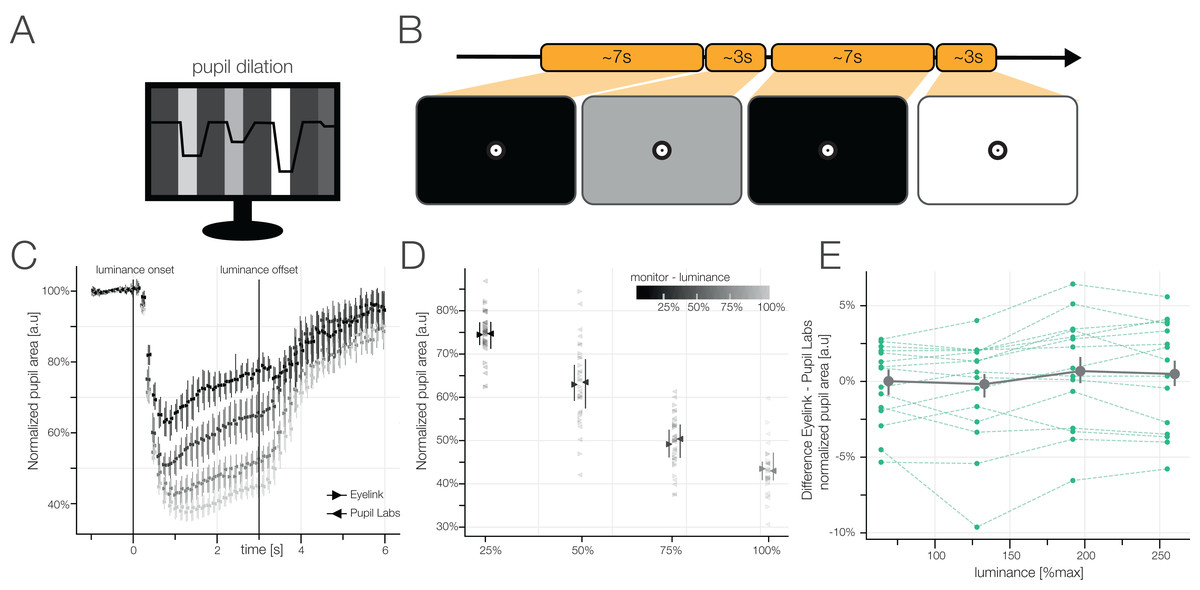Our pupil shrinks when it gets bright and opens up when it gets dark. We tested how well the eye tracker can measure this by presenting differently bright images interleaved by black images (so the pupil can go back (or near) to baseline). We found that on the average, both eye tracker give the same results (both eyetrackers are depicted on top of each other, barely differentiable in figure C) – but calculating the difference between eye trackers for each subject they differ quite a bit (green lines in E are not at 0%)!

## Take home

• Eye tracking scientists need information about the reliability and performance of their equipment to make informed decisions
• Every eye movement has their own parameters to check for, e.g. pupil dilation has different requirements to an eye tracker than microsaccade research

That concludes this short laypersons summary. If you have any question feel free to comment here, write me, Katharina Groß, or any of my other co-authors. (paper doi: https://doi.org/10.7717/peerj.7086 )

## Brain Art

I submitted this triptychon to the OHBM brain-art competition 2019. I used mouse myelin stains from the Brain Architecture Project and generated Rohrschach-Like creatures. I quite like how it turned out and I definitely want to do more in the future. I especially found the Allen Brain Atlas’ developmental mouse stains very cool – lots of potential there.

If you are interested in other art pieces related to neuroscience feel free to check out my thesis-art collection – one piece for each student I supervised.

Thanks to Ella Bosch & Anna Gert for latin & design advice!

## Threshold Free Cluster Enhancement explained

#### The multiple comparison problem

In neuroimaging analysis one often is confronted with many electrodes/voxels and many timepoints, and often performs a statistical test on each of these electrode/timepoint combinations. This leads to a massive multiple comparison problem, as the probability to find a false positive is greatly enhanced. In the following example we assume independence of all data points . For instance with only 10 electrodes/voxels and 10 timepoints and an alpha of 0.05, the probability for a false positive is:

$$p(significance^*|H_0) = 1-(1-0.05)^{10*10}=99.4$$

*significance of at least one sample

But electrodes/voxels and timepoints usually are not independent. Contrary, data is rather smooth over electrodes, voxels and time. Therefore, by combining data points close in space (electrodes / voxels) and time using so-called cluster tests, one can try to partially circumvent this problem.

For the IICCSSS Summer School I made some slides explaining multiple comparison correction (link to slides, pptx), including Threshold Free Cluster Enhancement (TFCE). I explained cluster permutation tests in an earlier blog post, which you might want to read before this one.

#### Why TFCE and not cluster permutation tests?

In order to use cluster permutation tests, one typically first calculates some kind of statistics, in our example student t-values for each datapoint over subjects (or trials for single subject analyses). The one has to specify a cluster-threshold which defines the clusters. This threshold might miss broad but “weak” clusters, and focus only on “strong” but peaky clusters.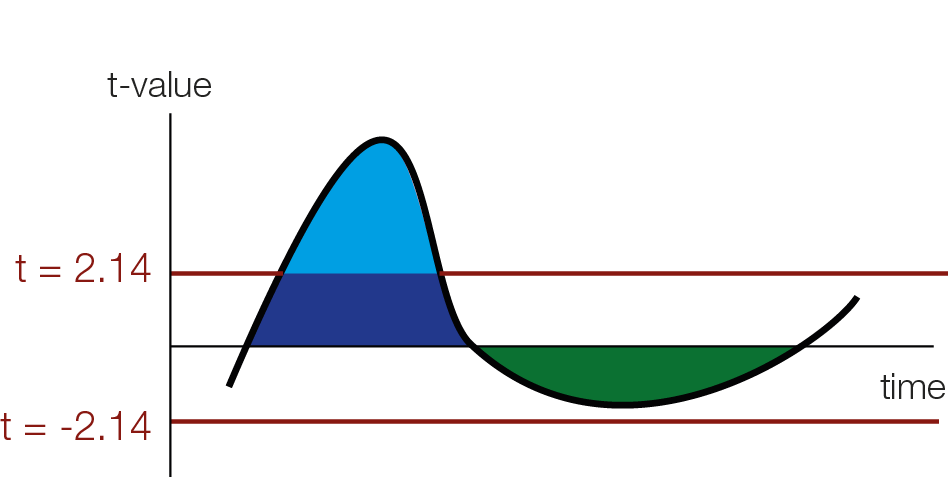As an example I depict students t-value of an effect over time. In this figure, the blue cluster will be detected (the thresholded cluster mass is depicted by the light blue area), but the broader green cluster would be missed

#### Threshold Free Cluster Enhancement

The intuition of TFCE is that we are going to try out all possible thresholds and see whether a given time-point belongs to a significant cluster under any of our set of cluster-thresholds. Instead of using cluster mass, we will use a weighted average between the cluster extend (e, how broad is the cluster, i.e. how many connected samples) and the cluster height (h, how high is the cluster, i.e. how large is the t-value / the evidence for an effect) according to the formula:

$$TFCE = \int_h e(h)^Eh^Hdh$$

for this blogpost, I will put the weights for the extend E and for the height H to 1 (therefore height ‘counts’ the same as width). The usual defaults are E=0.5 and H=2.Note that the weights E and H of the TFCE formula $e^E$ and $h^H$ are set to 1 and therefore ommitted in the gif. A non-gif version can be found in this powerpoint presentation: https://cloud.wirdreibei.de/s/GE6nwpRTrQdD76n (CC-By)

As you can see we use a discrete sum, approximating the integral from above. Another difference between TFCE and cluster permutations, is that you generate a TFCE value for each sample.

For instance a hypothetical t-value of 3 (red square in the above animation) is boosted by belonging to a cluster and might receive the TFCE value of 10. The resulting TFCE values can be thought of as a local scaling according to the “clusterdness” of a sample. Note that local minima and maxima stay at the same spot, this is different to a smoothing operation which could move the location of maxima and minima in time or space.

Because we calculated a TFCE value for each sample, we can also calculate a p-value for each sample. In order to get the p-values, we use the same trick wie used with cluster permutation test: the permutation part. We permute conditions (building the $H_0$), calculate the TFCE values for the permutated set, and take the max(TFCE) over all time points and electrodes/voxels. Our observed TFCE value then is either likely or unlikely given our empirical distribution of max TFCE values (under the $H_0$). But note that the interpretation is not that of a typical p-value at an electrodes/voxel!.

#### Interpretation of significant TFCE

I admit I made the following mistake before, it is a very convenient and easy mistake to make: As an example, let’s observe a significant sample e.g. at 100ms, using the TFCE procedure. This does not mean that the sample at 100ms shows a significant effect. It only means, that there exist at least one cluster-threshold (remember we tested all of them), where this sample belongs to a significant cluster. In other words, samples can be pushed to significance solely by being close to a “truely significant” cluster, without showing evidence by themselves to be significant.

I found this pretty confusing. But in practice it is important: Because we don’t know which samples make a cluster a significant one (all of them? half of them? only a single sample?) we cannot say much about the single sample, only about the cluster.

So, in practice what we do is that we look and report the p-values, but in addition make a descriptive statement on the cluster extend. For instance, you could argue that the t-values that you put in TFCE (or cluster permutation) are very much compatible with an effect from X ms to Y ms. Similar statements are also recommended on the fieldtrip site or in this recent paper by Jona Sassenhagen 2018.

Don’t write: “We found a significant cluster starting from 100ms to 200ms with a median effect of 5µV [3.5, 4.7µV].” or even “Conditions differed significantly from 100ms to 200ms (multiple comparison corrected)”.
Write: “We found a significant difference between conditions. The difference was driven by an effect from 100ms to 200ms with a median effect of 5µV [3.5, 4.7µV] .” or “We found a significant cluster, most compatible with an effect from 100ms to 200ms with a median effect of 5µV [3.5, 4.7µV] “.

Dont write: “At t=125ms the conditions differed significantly (TFCE correction for multiple comparisons) with a median effect of 5µV [3.5, 4.7µV] “
Write: “We found significant difference between conditions (TFCE correction for multiple comparisons). This difference was driven by a cluster starting at 125ms with a median effect of 5µV [3.5, 4.7µV] .”

These messages are much less snappy, sexy, short or easy to understand. The important bit is to signal to the reader that the cluster permutation test does not state significance about a single timepoint or electrode/voxel, but only indicates a significant difference somewhen/re between your conditions.

This problem has been recently discussed on twitter. One proposed alternative is All-Resolution-Inference. There is a barebone R-implementation in the hommel package and I would be interested in translating it to matlab to be readily usable with cluster permutation for EEG data.

Thanks for personal discussions (these are not endorsements, all mistakes in this blogpost are mine!) with Eelke Spaak, Robert Oostenveld, René Scheeringa, Olaf Dimigen & Phillip Alday + twitter interactions with Guillame A Rousselet, Cyril Pernet, Thomas Nichols and Martin Hebart. Thanks to Anna Lisa Gert for critical comments on this blogpost.

## Simulating EEG/ERP Data with SEREEGA & multiple comparison corrections

For the $i^2 c^2 s^3$ summer school I simulated quite a bit of data and analyzed them with several common multiple comparison methods. I used the SEREEGA toolbox for the simulation. All the MatLab-code can be found at the end of this post. In a follow-up blogpost I will extend the toolbox to continuous data that we can analyze with the unfold toolbox.

First, I simulated data based on three effects: Two early dipoles representing the P100, one right lateralized for the N170 and a deep one for the P300.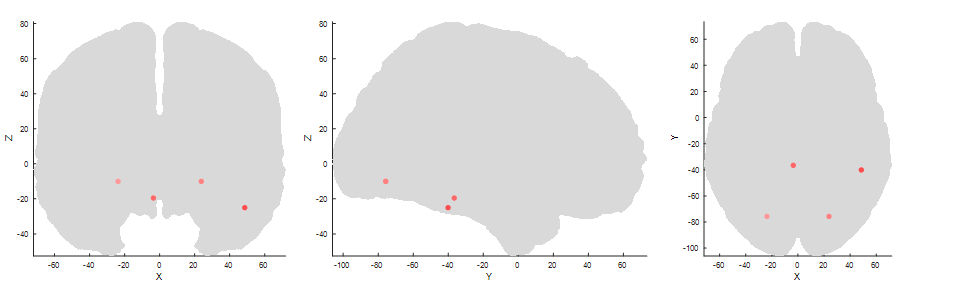Two symmetric sources for the P1 in the occipital cortex, one lateralized to the right cortex (third panel right most) for the N170 and a deep one, central for the P300.

I added brown and white noise to the simulated epochs. An exemplary eegplot shows that it kind of looks like EEG data.

I generate two conditions with two different condition-differences, one on the N170 and one on the P300 . The same data are depicted in the following three plots:

In the first row we see a butterfly plot of activation in all channels (the colored channels are depicted in the next plot). In the first topoplot-row we have the average activation and in the last row we have the condition differences.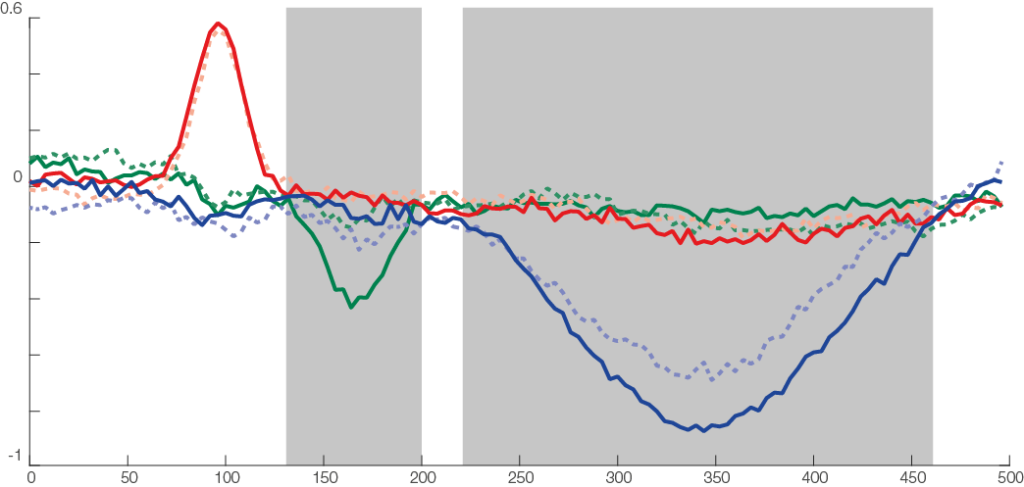The three types of components: a short but relatively strong (P100, red), a similar effect, short but strong (N170, condition difference) and a long effect (P300, with weak condition effect). Highlighted gray areas are approximate regions where the components showed condition differences. The noise was a mix between brown and white noise.

The red line is the P100, occipital effect. No difference between conditions. The green one is the N170, temporal effect. Only visible in one condition. The blue one is a P300 like, deep effect. Difference in amplitude between conditions.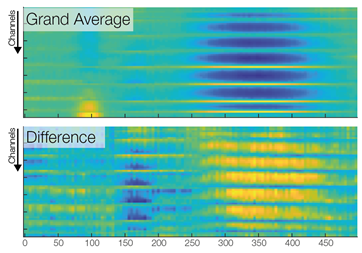A third way to look at the same data. Color indicates activation (blue => negative µV, yellow=> positive µV). Each row is one channel, each column one time point. Using such a plot, one looses spatial relations between the channels , but gains an nice overview of all the data. Note that the colorscales are different between the two panels.

Now we are ready to do some statistical testing and multiple comparison correction. I choose to compare uncorrected, bonferroni-holms, FDR (Benjamini-Hochberg), clustermass cluster permutation testing (LIMO toolbox) and threshold free cluster enhancement (EPT-TFCE toolbox).

In this instance, uncorrected p-value did not so bad, Bonferroni-Holms is, as expected, quite conservative. FDR hat troubles with the elongated cluster and TFCE/Cluster-permutation with the short one.

Note: This is only a single simulation. In order to move from these anectotal findings to proper statements, one would need to repeat the simulation 1000 times and see how often which samples were deemed significant (see Groppe 2011).

Thanks to Anna Lisa Gert for help with writing this post.

This is the code I used, it is a bit messy because it was programmed in a tutorial-style and I kept my debug-statements in there.

rng(1)
eeglab
end
% Threshold Free cluster enhancement
%% SEREEGA Data Generation
cfg = struct('debug',0)

if cfg.debug
end

%% set seed

%% noise
noise_brown = struct( ...
'type', 'noise', ...
'color', 'brown', ...
'amplitude', 2);
noise_brown = utl_check_class(noise_brown);

noise_white = struct( ...
'type', 'noise', ...
'color', 'white', ...
'amplitude', 2);
noise_white = utl_check_class(noise_white);

%% visual Cortex N170
vis = [];

vis.signal{1} = struct();
vis.signal{1}.peakLatency = 170;      % in ms, starting at the start of the epoch
vis.signal{1}.peakWidth = 100;        % in ms
vis.signal{1}.peakAmplitude = -5;      % in microvolt
vis.signal{1} = utl_check_class(vis.signal{1}, 'type', 'erp');
vis.signal{2} = noise_brown;

% right visual cortex
vis.source= lf_get_source_nearest(lf, [50 -40 -25]);
if cfg.debug
plot_source_location(vis.source, lf, 'mode', '3d','shrink',0);
end

% vis.orientation = utl_get_orientation_pseudotangential(vis.source,lf);
vis.orientationDv = [0 0 0]; % orientation does not change between trials
vis.orientation = [0.5,-0.46,1]; % I looked at the maps and found that this prientation most closely looked like a N170

if cfg.debug
%% This Code I used to generate random orientations and inspect the leadfield projections
for k = 1:10
vis.orientation = utl_get_orientation_random(1)
plot_source_projection(vis.source, lf, 'orientation', vis.orientation,'orientedonly',1);
%     title(vis.orientation)
end
end
%% Generate P100, take from SEREEGA Toolbox
p100 = utl_get_component_fromtemplate('visual_p100_erp',lf);
if cfg.debug
% Visualize the response
plot_signal_fromclass(vis.signal{1}, epochs);
end

%% Generate P300
p3 = [];

p3.signal{1} = struct();
p3.signal{1}.peakLatency = 350;      % in ms, starting at the start of the epoch
p3.signal{1}.peakWidth = 400;        % in ms
p3.signal{1}.peakAmplitude = -3;      % in microvolt
p3.signal{1} = utl_check_class(p3.signal{1}, 'type', 'erp');
p3.signal{2} = noise_brown;

% somewhere deep in the brain
p3.source= lf_get_source_nearest(lf, [0 -40 -25]);
if cfg.debug
%% visualize it using SEREEGA
plot_source_location(p3.source, lf, 'mode', '3d');
end

p3.orientation = utl_get_orientation_pseudotangential(p3.source,lf);
p3.orientationDv = [0 0 0];

p3.orientation = [0.03,-0.16,1];
if cfg.debug
%% again find an orientation that somehow matches P300topography
for k = 1:10
p3.orientation = utl_get_orientation_random(1)
plot_source_projection(p3.source, lf, 'orientation', p3.orientation,'orientedonly',1);
end
end

%% generate 25 random noise sources and combine all components
sources = lf_get_source_spaced(lf, 10, 25);
noise_brown.amplitude = 5;
comps = utl_create_component(sources, noise_brown, lf);
[comps1, comps2] = deal(comps);

% add P100 to noise components
comps1(end+1:end+2) = p100; %bilateral
comps2(end+1:end+2) = p100;

% N170 with condition difference
vis.signal{1}.peakAmplitude = -3;
comps1(end+1) = vis;
vis.signal{1}.peakAmplitude = -1;
comps2(end+1) = vis;

% broad, weak P300, with condition difference
p3.signal{1}.peakAmplitude = -10;
comps1(end+1) = p3;
p3.signal{1}.peakAmplitude = -12;
comps2(end+1) = p3;
%% Generate The EEG Data
epochs = struct();
epochs.n = 50;             % the number of epochs to simulate
epochs.srate = 250;        % their sampling rate in Hz
epochs.length = 500;

data1 = generate_scalpdata(comps1, lf, epochs);
data2 = generate_scalpdata(comps2, lf, epochs);
EEG1 = utl_create_eeglabdataset(data1, epochs, lf, 'marker', 'event1');
EEG2 = utl_create_eeglabdataset(data2, epochs, lf, 'marker', 'event2');
EEG = utl_reorder_eeglabdataset(pop_mergeset(EEG1, EEG2));

%% First inspection, this should match the intended topographies
pop_topoplot(EEG, 1, [0 100 170,300], '', [1 8]);
%% ERP-Like Figure
figure,
findtype = @(type)cellfun(@(x)strcmp(type,x),{EEG.event.type});

ploterp = @(chan)plot(EEG.times,...
[mean(squeeze(EEG.data(chan,:,findtype('event1'))),2),...
mean(squeeze(EEG.data(chan,:,findtype('event2'))),2)]);
figure,ploterp(44),title(EEG.chanlocs(44).labels)
hold on,ploterp(63),title(EEG.chanlocs(63).labels)
hold on,ploterp(30),title(EEG.chanlocs(30).labels)

%% Within-Subject T-Test using LIMO

[m,dfe,ci,sd,n,t,p] = limo_ttest(2,EEG.data(:,:,findtype('event1')),EEG.data(:,:,findtype('event2')),0.05);
%% simple imagesc plots
figure,subplot(2,1,1),imagesc(EEG.times,1:64,mean(EEG.data,3));box off
subplot(2,1,2),imagesc(EEG.times,1:64,m),box off
% Show data
figure
imagesc(m)

figure
imagesc(t)
%% Prepare statistics

%%
ept_tfce_nb = ept_ChN2(EEG.chanlocs,0); % the 1 to plot
tfce_res = ept_TFCE(...
permute(EEG.data(:,:,findtype('event1')),[3 1 2]),...
permute(EEG.data(:,:,findtype('event2')),[3,1,2]),EEG.chanlocs,'nperm',800,'flag_save',0,'ChN',ept_tfce_nb)

%%
t_h0 = nan([size(EEG.data,1),size(EEG.data,2),800]);
for b = 1:800
fprintf('%i/%i\n',b,800)
r_perm      = randperm(epochs.n*2); % Consider using Shuffle mex here (50-85% faster)...

nData       = EEG.data(:,:,r_perm);
sData{1}    = nData(:,:,1:epochs.n);
sData{2}    = nData(:,:,(epochs.n+1):(epochs.n*2));

t_h0(:,:,b) = (mean(sData{1},3)-mean(sData{2},3))./sqrt(var(sData{1},[],3)/epochs.n+var(sData{2},[],3)/epochs.n);

end
p_h0 = tpdf(t_h0,199);
%% Generate Cluster Permutation
limo_nb = limo_neighbourdist(EEG,50);
cluster_p(isnan(cluster_p(:))) = 1;
% F_tfce = limo_tfce(2,t.^2,limo_nb);

%% show significance methods
figure,
for k = 0:4
switch k
case 0
plot_p = p;
case 1
plot_p = bonf_holm(double(p),0.05);
case 2
[~,~,~,plot_p] = fdr_bh(p,0.05,'pdep','no'); % see groppe 2011 why pdep is fine

case 3
plot_p = cluster_p;

case 4
plot_p = tfce_res.P_Values;

end
plot_data= plot_p;
plot_data = log10(plot_data);
subplot(5,1,(k)+1)
eegvis_imagesc(m,t,'chanlocs',EEG.chanlocs,'times',EEG.times,...
%     caxis([-7,0])
%     if k ~=4
%         axis off
%     end
%     box off
box off
end

%%
hA = plot_topobutter(mean(EEG.data,3),EEG.times,EEG.chanlocs,'colormap',{'div'},'quality',40,'n_topos',12);

%%
figure
hA = plot_topobutter(cat(3,mean(EEG.data,3),m),EEG.times,EEG.chanlocs,'quality',32,'individualcolorscale','row','highlighted_channel',[44,63]);

plot_source_location([p100.source,vis.source,p3.source], lf, 'mode', '2d');



## Random Plot (constricting radial sinus)

This was the result of a (inconclusive) test of how the blind spot fills in things

theta = linspace(0, 2*pi, 50).';
KbName('UnifyKeyNames')
Factor = 10;
figure
while true

[X, Y] = meshgrid(linspace(-pi,pi,1000),linspace(-pi,pi,1000));
dist = sqrt((X.^2 + Y.^2));
imagesc(sin(dist*Factor))
colormap('gray'), axis equal
drawnow;
[~,keyCode] = KbWait;

kb = KbName(find(keyCode));
if ~iscell(kb)
switch kb
case 'LeftArrow'
Factor = 0.9*Factor;
case 'RightArrow'
Factor = 1.1*Factor;
case 'Esc'
continue
%         break
end
%                     display(kb)

end
end

## Thesis Art

I was a supervisor for Katharina Groß’s Bachelor’s Thesis. You can find the resulting paper (!) here on biorXiv

In her project we developed a new test battery to benchmark eye-trackers in many different tasks. We concurrently measured Pupil Labs Glasses and an Eyelink 1000. In the thesis art I selected six of the tasks and visualized the data of one subject using the letters of her thesis. The tasks I used are: Fixation Grid, Smooth Pursuit, Microsaccades, Blinks, Pupil Dilation and Free Viewing.

The idea of “thesis art” is to inspire discussion with persons who do not have an academic background or work in a different field. The thesis is hidden in the drawer, but the poster is out there at the wall for everyone to see. You can find all past thesis art pieces here

## No evidence for periodicity in reaction time histograms

### Introduction

In my last lab we discussed findings on the periodicity of reaction times (e.g. referred in Van Rullen 2003). These studies are usually old (Starting with Harter 1968, Pöppel 1968), with small N and not many trials. There was also a extensive discussion in the Max-Planck Journal “Naturwissenschaften” in the 90s (mostly in German, e.g. Vorberg & Schwarz 1987). A methodological critique is from Vorberg & Schwarz 1987. More discussion in Gregson (Gregson, Vorberg, Schwarz 1988). A new method to analyse periodicity is proposed by Jokeit 1990.

This is the newest research I could find on this topic.

### Analysing a large corpus of RT-data

I stumbled upon a large reaction time dataset (816 subjects, á 3370 trials, 2.3 million RTs)  from the English Lexicon Project (Balota et al 2007) and decided to look for these oscillations in reaction times .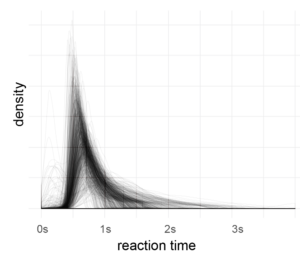After outlier correction (3*mad rule, see below), I applied a fourier-transformation on the histogram (1ms bins, accuracy of RT=1ms). Then I looked for peaks in the spectrum which are consistent over subjects.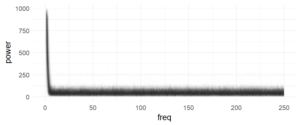Each subject is one line, no effect is visible here (in a log-scaled y-axis also no effect can be seen). The range (above ~7Hz) of the subject variance is roughly between +0 and 100

The following graph summarizes  the above graph (blue-smoother-curve = loess, span=0.1, each dot = mean over 800 subjects)):There are no peaks in the spectrum which I consider as consistent over subjects. I included higher frequencies (up to 250Hz) to get a visual estimate of the noise level (at such high frequencies, an effect seems utterly implausible). But of course, I’m ignoring  within subject information (i.e. a mixed model of some sort could be appropriate).

### Conclusions

In this large dataset, I cannot find periodicities of reaction time.

### Disclaimer

My approach may be too naive. I’m looking for more powerful ways to analyse these data. If you have an idea please leave a comment! I’m also not suggesting that the effects e.g. in Pöppels data are not real. Maybe there is a mistake in my analysis, I don’t know the data by heart, it might depend on the task employed …

### Thoughts

I had results like in Jokeit 1990 (but with 50Hz not with 100Hz), when I was using a bin-width of 5ms to 10ms bins.  The peak (in the figure with 6ms bins => 150hz) shifted depending on bin-size. I’m not perfectly sure, but I think it has to do with how integers are binned. In any case, if the effect is real and not an artefact of bin-width, it has to show up also with higher bin-sizes. Please note that Jokeit 1990 used a different methodology, he calculated the FFT on the histogram of reaction time **differences**.

I tried to use density estimates, but so far failed to get better results.

### Outlier plot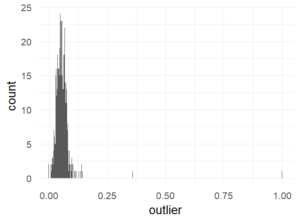Percentage of trials marked as outliers. This is well in the recommended range of 10% (Ratcliff).

### References

Bolota, D.A., Yap, M.J., Cortese, M.J., Hutchison, K.A., Kessler, B., Loftis, B., Neely, J.H., Nelson, D.L., Simpson, G.B., & Treiman, R. (2007). The English Lexicon Project. Behavior Research Methods, 39, 445-459. – http://elexicon.wustl.edu/about.asp

library(ggplot2)
library(dplyr)
theme_set(theme_minimal(20))

d$Sub_ID = factor(d$Sub_ID)
d$D_RT = as.integer(d$D_RT)

d = d%>%group_by(Sub_ID)%>%mutate(outlier = abs(D_RT-median(D_RT))>3*mad(D_RT,na.rm = T))
d$outlier[d$D_RT<1] = TRUE
d$outlier[is.na(d$D_RT)] = TRUE

# outlier plot
ggplot(d%>%group_by(Sub_ID)%>%summarise(outlier=mean(outlier)),aes(x=outlier))+geom_histogram(binwidth = 0.001)

fft_on_hist = function (inp){
maxT = 4
minT = 0
fs = 1000

h = hist(inp$D_RT,breaks = 1000*seq(minT,maxT,1/fs),plot = F) h = h$counts;

# I tried to use density estimates instead of histograms, but it was difficult
#h = density(inp$D_RT,from = minT,to=4000,n=4000) #h = h$y
f = fft(h)
f = abs(f[seq(1,length(f)/2)])

return(data.frame(power = f, freq = seq(0,fs/2-1/maxT,1/maxT)))
}

d_power = d%>%subset(outlier==F)%>%group_by(Sub_ID)%>%do(fft_on_hist(.))

ggplot(d_power,aes(x=freq,y=(power),group=Sub_ID))+geom_path(alpha=0.01)
ggplot(d_power,aes(x=freq,y=log10(power)))+geom_path(alpha=0.01)

ggplot(d_power%>%group_by(freq)%>%summarise(power=mean(power)),aes(x=freq,y=(power)))+geom_point()+stat_smooth(method='loess',span=0.1,se=F,size=2)+xlim(c(10,250))+ ylim(c(47,53))



1 of 5
12345